Test: Frequency - Domain Analysis

# Test: Frequency - Domain Analysis

Test Description

## 20 Questions MCQ Test GATE Electrical Engineering (EE) 2023 Mock Test Series | Test: Frequency - Domain Analysis

Test: Frequency - Domain Analysis for Electronics and Communication Engineering (ECE) 2023 is part of GATE Electrical Engineering (EE) 2023 Mock Test Series preparation. The Test: Frequency - Domain Analysis questions and answers have been prepared according to the Electronics and Communication Engineering (ECE) exam syllabus.The Test: Frequency - Domain Analysis MCQs are made for Electronics and Communication Engineering (ECE) 2023 Exam. Find important definitions, questions, notes, meanings, examples, exercises, MCQs and online tests for Test: Frequency - Domain Analysis below.
Solutions of Test: Frequency - Domain Analysis questions in English are available as part of our GATE Electrical Engineering (EE) 2023 Mock Test Series for Electronics and Communication Engineering (ECE) & Test: Frequency - Domain Analysis solutions in Hindi for GATE Electrical Engineering (EE) 2023 Mock Test Series course. Download more important topics, notes, lectures and mock test series for Electronics and Communication Engineering (ECE) Exam by signing up for free. Attempt Test: Frequency - Domain Analysis | 20 questions in 60 minutes | Mock test for Electronics and Communication Engineering (ECE) preparation | Free important questions MCQ to study GATE Electrical Engineering (EE) 2023 Mock Test Series for Electronics and Communication Engineering (ECE) Exam | Download free PDF with solutions
 1 Crore+ students have signed up on EduRev. Have you?
Test: Frequency - Domain Analysis - Question 1

### An under damped second order system having a transfer function of the form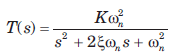has a frequency response plot shown in fig.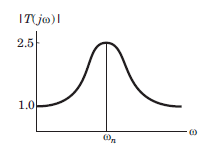Q. The system gain K is

Detailed Solution for Test: Frequency - Domain Analysis - Question 1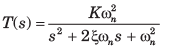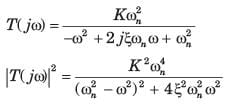From the fig. P6.5.1-2, |T(j0)| = 1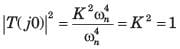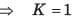Test: Frequency - Domain Analysis - Question 2

### An under damped second order system having a transfer function of the formhas a frequency response plot shown in fig.Q. The damping factor ξ is approximately

Detailed Solution for Test: Frequency - Domain Analysis - Question 2

The peak value of T(jω) occurs when the denominator of function |T(jω)|2 is minimum i.e. when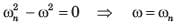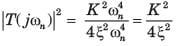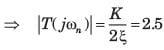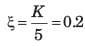Test: Frequency - Domain Analysis - Question 3

### Consider the Bode plot of a ufb system shown in fig.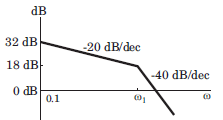Q. The steady state error corresponding to a rampinput is

Detailed Solution for Test: Frequency - Domain Analysis - Question 3

The Bode plot is as shown in fig.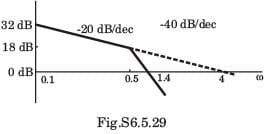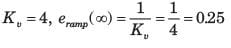Test: Frequency - Domain Analysis - Question 4

Consider the Bode plot of a ufb system shown in fig.The damping ratio is

Detailed Solution for Test: Frequency - Domain Analysis - Question 4

From Fig.: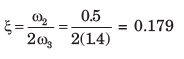Test: Frequency - Domain Analysis - Question 5

The Nyquist plot of a open-loop transfer function G(jω)H(jω) of a system encloses the (-1, j0) point. The gain margin of the system is

Detailed Solution for Test: Frequency - Domain Analysis - Question 5

If Nyquist plot encloses the point (-1, j0), the system is unstable and gain margin is negative.

Test: Frequency - Domain Analysis - Question 6

Consider a ufb system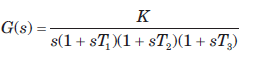The angle of asymptote, which the Nyquist plotapproaches as ω → 0 is

Detailed Solution for Test: Frequency - Domain Analysis - Question 6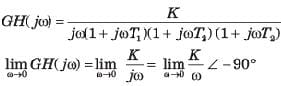Hence, the asymptote of the Nyquist plot tends to an angle of -90° as ω→ 0.

Test: Frequency - Domain Analysis - Question 7

If the gain margin of a certain feedback system isgiven as 20 dB, the Nyquist plot will cross the negativereal axis at the point

Detailed Solution for Test: Frequency - Domain Analysis - Question 7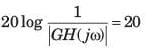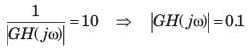Since system is stable, it will cross at s = -0.1

Test: Frequency - Domain Analysis - Question 8

The transfer function of an open-loop system is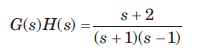The Nyquist plot will be of the form

Detailed Solution for Test: Frequency - Domain Analysis - Question 8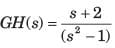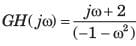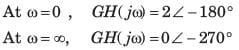Hence (B) is correct option.

Test: Frequency - Domain Analysis - Question 9

Consider a ufb system whose open-loop transfer function is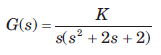The Nyquist plot for this system is

Detailed Solution for Test: Frequency - Domain Analysis - Question 9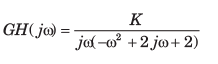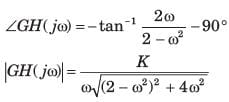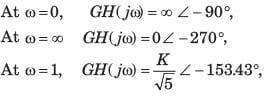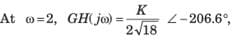Due to s there will be a infinite semicircle. Hence (C) is correct option.

Test: Frequency - Domain Analysis - Question 10

The open loop transfer function of a system is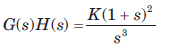The Nyquist plot for this system is

Detailed Solution for Test: Frequency - Domain Analysis - Question 10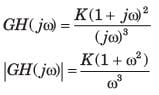∠GH(jw) = -270°+ 2 tan-1ω
For ω = 0, GH(jω) = ∞∠ -270°
For ω = 1 , ∠GH(jω) =  -180°
For ω = ∞, GH(jω) = 0 ∠ - 90°
As ω increases from 0 to ∞, phase goes -270° to -90°.
Due to s3 term there will be 3 infinite semicircle.

Test: Frequency - Domain Analysis - Question 11

For the certain unity feedback system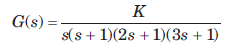The Nyquist plot is

Detailed Solution for Test: Frequency - Domain Analysis - Question 11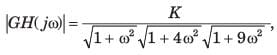∠GH(j(ω) = -90 °- tan-1 ω - tan-1 2ω - tan -1 3ω,
For ω = 0, GH(jω) = ∞∠ - 90°,
For ω = ∞, GH(jω) = 0∠- 360°,
Hence (A) is correct option.

Test: Frequency - Domain Analysis - Question 12

The Nyquist plot of a system is shown in fig. The open-loop transfer function is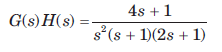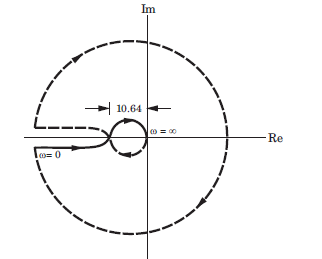The no. of poles of closed loop system in RHP are

Detailed Solution for Test: Frequency - Domain Analysis - Question 12

The open-loop poles in RHP are P = 0. Nyquist path enclosed 2 times the point (-1 + j0). Taking clockwise encirclements as negative N = -2.
N = P - Z, -2 = 0 -Z , Z = 2 which implies that two poles of closed-loop system are on RHP.

Test: Frequency - Domain Analysis - Question 13

The open-loop transfer function of a feedback control system is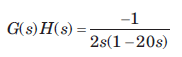Q. The Nyquist plot for this system is

Detailed Solution for Test: Frequency - Domain Analysis - Question 13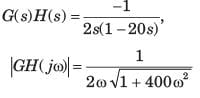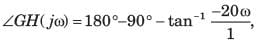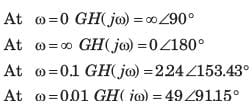Test: Frequency - Domain Analysis - Question 14

If the damping of the system becomes equal to zero, which condition of the resonant frequency is likely to occur?

Test: Frequency - Domain Analysis - Question 15

A unity feedback system has the open loop transfer function G(s)=1/((s−1)(s+2)(s+3))
The Nyquist plot of GG encircle the origin

Test: Frequency - Domain Analysis - Question 16

The open-loop transfer function of a feedback system is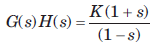Q. The Nyquist plot of this system is

Detailed Solution for Test: Frequency - Domain Analysis - Question 16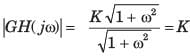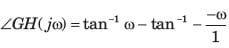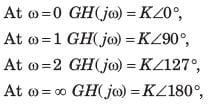Test: Frequency - Domain Analysis - Question 17

The open-loop transfer function of a feedback system isThe system is stable for K

Detailed Solution for Test: Frequency - Domain Analysis - Question 17

RHP poles of open-loop system P = 1, Z = P - N .
For closed loop system to be stable, Z = 0, 0 =1 -  N ⇒N = 1
There must be one anticlockwise rotation of point (-1+ j0). It is possible when K > 1.

Test: Frequency - Domain Analysis - Question 18

A unity feedback system has open-loop transfer function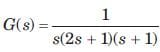Q. The Nyquist plot for the system is

Detailed Solution for Test: Frequency - Domain Analysis - Question 18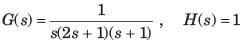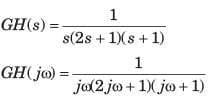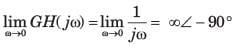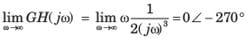The intersection with the real axis can be calculated as {GH(jω)} = 0, The condition gives ω(2ω2 -1) = 0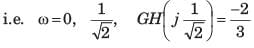With the above information the plot in option (C) is correct.

Test: Frequency - Domain Analysis - Question 19

A unity feedback system has open-loop transfer function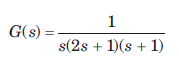Q. The phase crossover and gain crossover frequenciesare

Detailed Solution for Test: Frequency - Domain Analysis - Question 19

The Nyquist plot crosses the negative real axis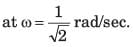Hence phase crossover frequency is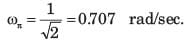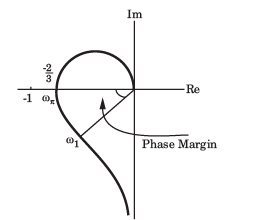The frequency at which magnitude unity is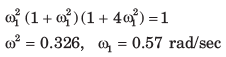Test: Frequency - Domain Analysis - Question 20

A unity feedback system has open-loop transfer functionThe gain margin and phase margin are

Detailed Solution for Test: Frequency - Domain Analysis - Question 20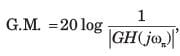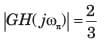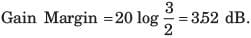∠GH(jω) = -90°- tan-1 ω- tan-1 2ω ,
At unit gain ω1 = 0.57 rad/sec,
Phase at this frequency is ∠GH(jω1) = -90°- tan-1 0.57 -tan-1 2(0.57) = -168.42°
Phase margin = -168.420+180° = 11.6°
Note that system is stable. So gain margin and phase margin are positive value. Hence only possible option is (D).

## GATE Electrical Engineering (EE) 2023 Mock Test Series

22 docs|274 tests
 Use Code STAYHOME200 and get INR 200 additional OFF Use Coupon Code
Information about Test: Frequency - Domain Analysis Page
In this test you can find the Exam questions for Test: Frequency - Domain Analysis solved & explained in the simplest way possible. Besides giving Questions and answers for Test: Frequency - Domain Analysis, EduRev gives you an ample number of Online tests for practice

## GATE Electrical Engineering (EE) 2023 Mock Test Series

22 docs|274 tests